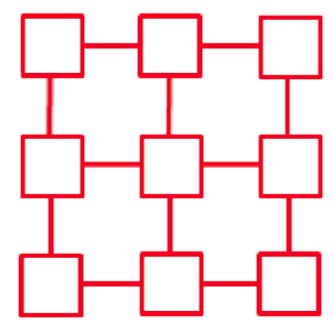#### You may also like### Consecutive Numbers

An investigation involving adding and subtracting sets of consecutive numbers. Lots to find out, lots to explore.### Roll These Dice

Roll two red dice and a green dice. Add the two numbers on the red dice and take away the number on the green. What are all the different possible answers?### Domino Square

Use the 'double-3 down' dominoes to make a square so that each side has eight dots.

# Number Differences

##### Age 7 to 11Challenge Level

You might like to try A Ring of Numbers and More Rings of Numbers before this problem.

Place the numbers from 1 to 9 in the squares below so that the difference between joined squares is odd. (You must use each of the numbers once.)Can you find some other ways to do this? Explain how you do this.

Can you put the numbers in the squares so that the difference between joined squares is even?